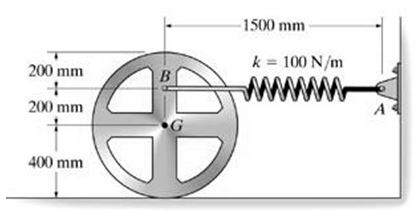# The 32-kg wheel has a radius of gyration about its center of gravity G of kG = 250 mm. The spring...

## Question:

The 32-kg wheel has a radius of gyration about its center of gravity G of kG = 250 mm. The spring AB has a stiffness k = 100 N/m and an unstretched length of 500 mm. The wheel is released from rest. If the wheel rolls without slipping, determine its angular velocity, measured clockwise when it has rotated clockwise 90{eq}^\circ {/eq} from the position shown.## Conservation of energy:

Energy conservation is a continuous process and it is conserved. Loss of energy takes place because it is converted into other forms, like heat and sound. Friction is one of the cause of energy loss.

Given

• Mass is {eq}m = 32\;{\rm{kg}}. {/eq}
• Stiffness is {eq}k = 100\;{{\rm{N}} {\left/ {\vphantom {{\rm{N}} {\rm{m}}}} \right. } {\rm{m}}}. {/eq}
• Unstretched length is {eq}l = 500\;{\rm{mm}}. {/eq}

Applying conversion of energy we get,

{eq}\begin{align*} {\rm{Initial}}\;{\rm{energy}} &= {\rm{Final}}\;{\rm{energy}}\\ {K_1} + {V_1} &= {K_2} + {V_2}......\left( 1 \right) \end{align*} {/eq}

Here, {eq}{K_1},{K_2} {/eq} are kinetic energy and {eq}{V_1}, {V_2} {/eq} are spring energy.

As wheel is released from rest so,

{eq}{K_1} = 0 {/eq}

And {eq}{V_1} = \dfrac{1}{2}kx_1^2 {/eq}

Here, {eq}{x_1} {/eq} is stretched length of spring at initial which is calculated as,

{eq}\begin{align*} {x_1} &= 1500 - 500\\ &= 1000\;{\rm{mm}} \end{align*} {/eq}

Therefore {eq}{V_1} {/eq} is calculated as,

{eq}\begin{align*} {V_1} &= \dfrac{1}{2}kx_1^2\\ &= \dfrac{1}{2} \times 100 \times 1\\ &= 50\;{\rm{Nm}} \end{align*} {/eq}

And kinetic energy at final position will be,

{eq}\begin{align*} {K_2} &= \dfrac{1}{2}I{\omega ^2} + \dfrac{1}{2}mv_2^2\\ &= \dfrac{1}{2}\left( {32 \times {{0.25}^2}} \right){\omega ^2} + \dfrac{1}{2} \times 32 \times {\left( {0.4\omega } \right)^2}\\ &= 3.56{\omega ^2} \end{align*} {/eq}

And the spring energy will be given by,

{eq}{V_2} = \dfrac{1}{2}kx_2^2 {/eq}

Here, {eq}{x_2} {/eq} is stretched length in final position.

So displacement during {eq}90^\circ rotation {/eq} will be ,

{eq}\begin{align*} d &= rv\\ &= 0.4 \times \dfrac{\pi }{2}\\ &= 0.2\pi \end{align*} {/eq}

Displacement of point {eq}B {/eq} after rotation is calculated as,

{eq}\begin{align*} {{x'}_2} &= 1.5 - 0.2 - 0.2\pi \\ &= 0.6717\;{\rm{m}} \end{align*} {/eq}

Stretched length at final point will be,

{eq}\begin{align*} {x_2} &= \sqrt {{{\left( {0.671} \right)}^2} + {{\left( {0.2} \right)}^2}} \\ &= 0.2008\;{\rm{m}} \end{align*} {/eq}

So spring energy will be,

{eq}\begin{align*} {V_2} &= \dfrac{1}{2} \times 100 \times {\left( {0.2008} \right)^2}\\ 7&= 2.016\;{\rm{Nm}} \end{align*} {/eq}

Substituting values in equation {eq}\left( 1 \right) {/eq}

{eq}\begin{align*} {K_1} + {V_1} &= {K_2} + {V_2}\\ 0 + 50 &= 3.56\omega + 2\\ \omega &= 3.67\;{{{\rm{rad}}} {\left/ {\vphantom {{{\rm{rad}}} {{\rm{sec}}}}} \right. } {{\rm{sec}}}} \end{align*} {/eq}

Thus, angular velocity is {eq}\omega = 3.67\;{{{\rm{rad}}} {\left/ {\vphantom {{{\rm{rad}}} {{\rm{sec}}}}} \right. } {{\rm{sec}}}} {/eq}